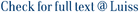Stochastic optimal control problems governed by delay equations with delay in the control are usually more difficult to study than the the ones when the delay appears only in the state. This is particularly true when we look at the associated Hamilton-Jacobi-Bellman (HJB) equation. Indeed, even in the simplified setting (introduced first by Vinter and Kwong for the deterministic case) the HJB equation is an infinite dimensional second order semilinear Partial Differential Equation (PDE) that does not satisfy the so-called "structure condition" which substantially means that "the noise enters the system with the control." The absence of such condition, together with the lack of smoothing properties which is a common feature of problems with delay, prevents the use of the known techniques (based on Backward Stochastic Differential Equations (BSDEs) or on the smoothing properties of the linear part) to prove the existence of regular solutions of this HJB equation and so no results on this direction have been proved till now. In this paper we provide a result on existence of regular solutions of such kind of HJB equations and we use it to solve completely the corresponding control problem finding optimal feedback controls also in the more difficult case of pointwise delay. The main tool used is a partial smoothing property that we prove for the transition semigroup associated to the uncontrolled problem. Such results holds for a specific class of equations and data which arises naturally in many applied problems.

Stochastic Optimal Control with Delay in the Control: solution through partial smoothing / Gozzi, Fausto; Masiero, Federica. - 1506.06013:(2015).

### Stochastic Optimal Control with Delay in the Control: solution through partial smoothing

#### Abstract

Stochastic optimal control problems governed by delay equations with delay in the control are usually more difficult to study than the the ones when the delay appears only in the state. This is particularly true when we look at the associated Hamilton-Jacobi-Bellman (HJB) equation. Indeed, even in the simplified setting (introduced first by Vinter and Kwong for the deterministic case) the HJB equation is an infinite dimensional second order semilinear Partial Differential Equation (PDE) that does not satisfy the so-called "structure condition" which substantially means that "the noise enters the system with the control." The absence of such condition, together with the lack of smoothing properties which is a common feature of problems with delay, prevents the use of the known techniques (based on Backward Stochastic Differential Equations (BSDEs) or on the smoothing properties of the linear part) to prove the existence of regular solutions of this HJB equation and so no results on this direction have been proved till now. In this paper we provide a result on existence of regular solutions of such kind of HJB equations and we use it to solve completely the corresponding control problem finding optimal feedback controls also in the more difficult case of pointwise delay. The main tool used is a partial smoothing property that we prove for the transition semigroup associated to the uncontrolled problem. Such results holds for a specific class of equations and data which arises naturally in many applied problems.
##### Scheda breve Scheda completa Scheda completa (DC)Stochastic Optimal Control with Delay in the Control: solution through partial smoothing / Gozzi, Fausto; Masiero, Federica. - 1506.06013:(2015).
File in questo prodotto:
Non ci sono file associati a questo prodotto.
##### Pubblicazioni consigliate

Caricamento pubblicazioni consigliate

I documenti in IRIS sono protetti da copyright e tutti i diritti sono riservati, salvo diversa indicazione.

Utilizza questo identificativo per citare o creare un link a questo documento: `http://hdl.handle.net/11385/159846`
##### Attenzione

Attenzione! I dati visualizzati non sono stati sottoposti a validazione da parte dell'ateneo

##### Citazioni
•ND
•ND